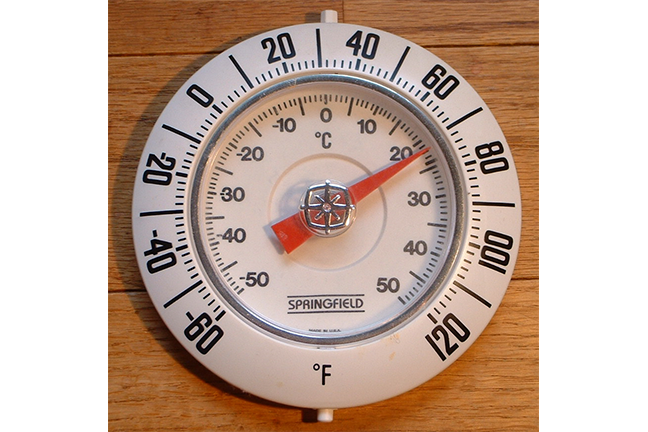1.16

## UNSW Sydney22 C = 71 F

# Fahrenheit to Celsius conversion

How hot is it today? Well, that depends where you are — in more ways than one. If you are in a hot country, the temperature is higher, and in a cold country the temperature is lower. Okay, so what else is new? Well there is also the issue of how we measure temperature.

In this step we have a look at the important conversion between the Celsius and Fahrenheit systems, illustrating the idea of a linear relation which is not a direct proportionality.

## Celsius versus Fahrenheit

In most countries around the world, temperature is measured in the Celsius system, where water freezes at $\normalsize{0}$ and boils at $\normalsize{100}$. Such countries include those in Europe, most of Asia and Africa, and Australia. However there are some countries, including notably the USA, where the Fahrenheit system is used, with water freezing at $\normalsize{32}$ and boiling at $\normalsize{212}$. Canada uses a mix of Celsius and Fahrenheit.

So a natural question is: how do we convert from one temperature to another? One way is with a chart:

Celsius Fahrenheit
0 32
10 50
20 68
30 86
40 104
50 122
60 140
70 158
80 176
90 194
100 212

A more visual way is to plot corresponding points. Let’s denote the two variables by $\normalsize{F}$ and $\normalsize{C}$, standing (of course) for Fahrenheit and Celsius respectively.

## A linear relationship

The value $\normalsize{F=32}$ goes with $\normalsize{C=0}$, while the value $\normalsize{F=212}$ goes with the value $\normalsize{C=100}$. So let’s make a graph.

Suppose we want primarily to convert from Celsius values to Fahrenheit. Then we would treat $\normalsize{C}$ as the horizontal variable, and $\normalsize{F}$ as the vertical variable. Here is then our $\normalsize{C-F}$ plane, with the two points that we know:For the line $\normalsize{F=mC+b}$, the $\normalsize{F}$-intercept is $\normalsize{b}$, which we can see is $\normalsize{32}$. How about the slope of the line? It is

So

## Converting temperatures

Let’s get some practice converting from one system to the other. You can use the formula, or possibly eyeball things from the graph of the line.

Q1 (E): (room temperature) So using our formula, find $\normalsize{F}$ when $\normalsize{C=22}$.

Q2 (E): (hot day) Find $\normalsize{F}$ when $\normalsize{C=30}$.

Q3 (E): (cold day) Find $\normalsize{F}$ when $\normalsize{C=-10}$.© “Sahara Desert” by wonker/Flickr CC BY 2.0

Q4 (E): (hottest day on record) Find $\normalsize{F}$ when $\normalsize{C=56.7}$ (recorded on 10 July 1913 at Greenland Ranch, Death Valley, California, USA).© “Talkessel von Werchojansk” Public Domain/Wikimedia Commons

Q5 (M): (coldest day on record) When $\normalsize{F=−128.6}$ find $\normalsize{C}$ (recorded on 21 July 1983 at the Soviet Vostok Station in Antarctica).

## Fahrenheit to Celsius

Q6 (M): Rearrange the equation ${\normalsize F=\frac{9}{5}C+32}$ to find $\normalsize{C}$ in terms of $\normalsize{F}$.A1. (room temperature) $\normalsize{F=71.6}$

A2. (hot day) $\normalsize{F=86}$

A3. (cold day) $\normalsize{F=14}$

A4. (hottest day) $\normalsize{F=134}$

A5. (coldest day) $\normalsize{C=-89.2}$

A6. You can (if you already know how) construct the line using the two points on the graph. A faster method is to rearrange the equation ${\normalsize F = \frac{9}{5}C + 32}$ into

We could also rewrite this as